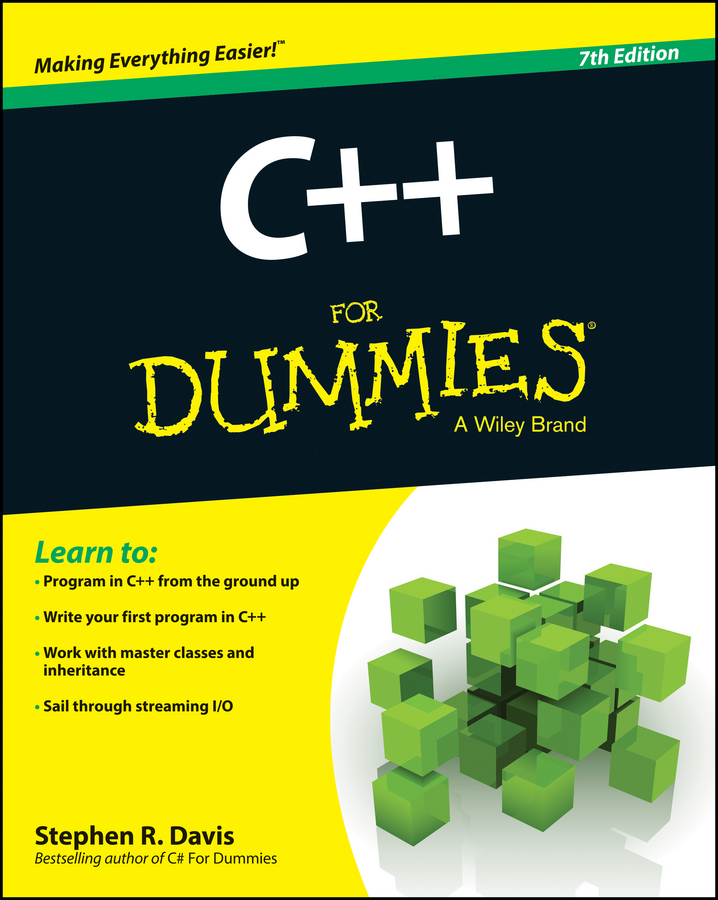##### C++ For DummiesC++ variables are stored internally as so-called binary numbers. Binary numbers are stored as a sequence of 1 and 0 values known as bits. Most of the time, you don't really need to deal with numbers at the bit level; however, there are occasions when doing so is convenient. C++ provides a set of operators for this purpose.

The so-called bitwise logical operators operate on their arguments at the bit level. To understand how they work, examine how computers store variables.

## The decimal number system

The numbers that you are familiar with are known as decimal numbers because they are based on the number 10. In general, the programmer expresses C++ variables as decimal numbers. Thus, you would say that the value of var is 123, for example.

A number such as 123 refers to 1 * 100 + 2 * 10 + 3 * 1. Each of these base numbers — 100, 10, and 1 — is a power of 10.

123 = 1 * 100 + 2 * 10 + 3 * 1

Expressed in a slightly different but equivalent way:

123 = 1 * 102 + 2 * 101 + 3 * 100

Remember that any number to the zero power is 1.

## Other number systems

The use of a base number of 10 for the counting system stems, in all probability, from the fact that humans have 10 fingers, the original counting tools. The alternative would have been base 20.

If dogs had invented our numbering scheme, it may well have been based on the numeral 8 (one digit of each paw is out of sight on the back part of the leg). Such an octal system would have worked just as well:

12310 = 1 * 82 + 7 * 81 + 3 * 80 = 1738

The small 10 and 8 here refer to the numbering system, 10 for decimal (base 10) and 8 for octal (base 8). A counting system may use any positive base.

## The binary number system

Computers have essentially two fingers. (Maybe that's why computers are so stupid: Without an opposable thumb, they can't grasp anything. And then again, maybe not.) Computers prefer counting using base 2. The number 12310 would be expressed as:

12310 = 0*128 + 1*64 + 1*32 + 1*16 + 1*8 + 0*4 +1*2 + 1*1
= 011110112

It is always convention to express binary numbers by using 4, 8, 32, or 64 binary digits even if the leading digits are zero. This is also because of the way computers are built internally.

Because the term digit refers to a multiple of ten, a binary digit is called a bit. The term stems from binary (b-) digit (-it). Eight bits make up a byte. A word is usually either two or four bytes.

With such a small base, it is necessary to use a large number of bits to express numbers. It is inconvenient to use an expression such as 011110112 to express such a mundane value as 12310. Programmers prefer to express numbers by units of bytes, or eight bits.

A single, four-bit digit is essentially base 16, because four bits can express up to any value from 0 to 15. Base 16 is known as the hexadecimal counting system. Hexadecimal is often contracted to simply hex.

Hexadecimal uses the same digits for the numbers 0 through 9. For the digits between 9 and 16, hexadecimal uses the first six letters of the alphabet: A for 10, B for 11, and so on. Thus, 12310 becomes 7B16.

123 = 7 * 161 + B (i.e., 11) * 160 = 7B16

Because programmers prefer to express numbers in 4, 8, 32, or 64 bits, they similarly prefer to express hexadecimal numbers in 1, 2, 4, or 8 hexadecimal digits even when the leading digits are 0.

Finally, it is inconvenient to express a hexadecimal number such as 7B16 using a subscript, because terminals don't support subscripts. Even on a word processor, it is inconvenient to change fonts to and from subscript mode just to type two digits. Therefore, programmers use the convention of beginning a hexadecimal number with a 0x (the reason for such a strange conviction goes back to the early days of C). Thus, 7B becomes 0x7B. Using this convention, 0x7B is equal to 123 (while 0x123 is equal to 291.)

All of the mathematical operators can be performed on hexadecimal numbers in the same way that they are applied to decimal numbers. The reason that we can't perform a multiplication such as 0xC * 0xE in our heads has more to do with the multiplication tables we learned in school than on any limitation in the number system.